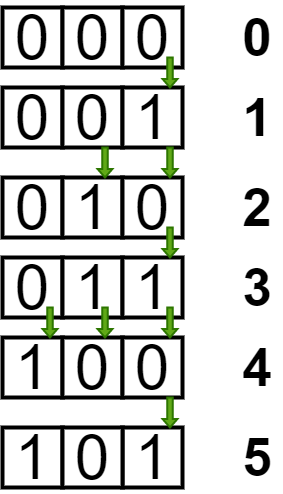# Sum of Hamming difference of consecutive numbers from 0 to N | Set 2

• Difficulty Level : Medium
• Last Updated : 12 May, 2021

Given a number N, the task is to find the sum of Hamming difference of consecutive numbers from 0 to N.

Hamming Distance between two integers is the number of bits which are different at the same position in both numbers.

Examples:

Input:
Output:
Explanation:
Difference between (0, 1) = 1, (1, 2) = 2,
(2, 3) = 1, (3, 4) = 3, (4, 5) = 1.
So the total sum is 1 + 2 + 1 + 3 + 1 = 8
Input:
Output: 16

Naive and Logarithmic Approach: Refer to Sum of bit differences for numbers from 0 to N for the naive approach and a logarithmic approach to calculate sum based on checking whether N as powers of 2 or not.
Approach: In this article, an approach based on observing the number of changes occurring in all the bits starting from the LSB to the MSB is discussed.
Follow the steps below to solve the problem:

• It can be observed that the Least Significant Bit will change N times. The 2nd Least significant bit will change floor(N/2) times (i.e (N – 1)/2 if N is odd and N/2 if N is even). Hence, the ith bit will change floor(N/2i) times.• Hence, to solve this problem we can simply store the sum

N + floor(N/2) + … floor(N/2i)

• until the term does not become zero.

Below is the implementation of the above approach:

## C++

 `// C++ Program to implement``// the above approach` `#include ``using` `namespace` `std;` `// Function to calculate and``// return the hamming distance``// between all consecutive``// numbers from 0 to N``int` `TotalHammingDistance(``int` `n)``{``    ``int` `i = 1, sum = 0;``    ``while` `(n / i > 0) {``        ``sum = sum + n / i;``        ``i = i * 2;``    ``}``    ``return` `sum;``}` `// Driver Code``int` `main()``{``    ``int` `N = 9;``    ``cout << TotalHammingDistance(N);``    ``return` `0;``}`

## Java

 `// Java program to implement the``// above approach``import` `java.util.*;` `class` `GFG{` `// Function to calculate and``// return the hamming distance``// between all consecutive``// numbers from 0 to N``static` `int` `TotalHammingDistance(``int` `n)``{``    ``int` `i = ``1``, sum = ``0``;``    ``while` `(n / i > ``0``)``    ``{``        ``sum = sum + n / i;``        ``i = i * ``2``;``    ``}``    ``return` `sum;``}` `// Driver code``public` `static` `void` `main(String[] args)``{``    ``int` `N = ``9``;``    ` `    ``System.out.println(TotalHammingDistance(N));``}``}` `// This code is contributed by offbeat`

## Python3

 `# Python3 program to implement``# the above approach` `# Function to calculate and``# return the hamming distance``# between all consecutive``# numbers from 0 to N``def` `TotalHammingDistance(n):``    ` `    ``i ``=` `1``    ``sum` `=` `0``    ` `    ``while` `(n ``/``/` `i > ``0``):``        ``sum` `=` `sum` `+` `n ``/``/` `i``        ``i ``=` `i ``*` `2``        ` `    ``return` `sum` `# Driver Code``if` `__name__ ``=``=` `'__main__'``:``    ` `    ``N ``=` `9``    ` `    ``print``(TotalHammingDistance(N))` `# This code is contributed by mohit kumar 29`

## C#

 `// C# Program to implement``// the above approach``using` `System;``class` `GFG{` `// Function to calculate and``// return the hamming distance``// between all consecutive``// numbers from 0 to N``static` `int` `TotalHammingDistance(``int` `n)``{``    ``int` `i = 1, sum = 0;``    ``while` `(n / i > 0)``    ``{``        ``sum = sum + n / i;``        ``i = i * 2;``    ``}``    ``return` `sum;``}` `// Driver Code``public` `static` `void` `Main()``{``    ``int` `N = 9;``    ``Console.Write(TotalHammingDistance(N));``}``}` `// This code is contributed by Code_Mech`

## Javascript

 ``

Output:

`16`

Time Complexity: O(logN)
Auxiliary Space: O(1)

My Personal Notes arrow_drop_up